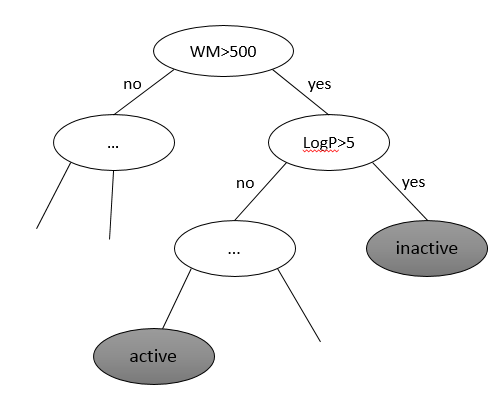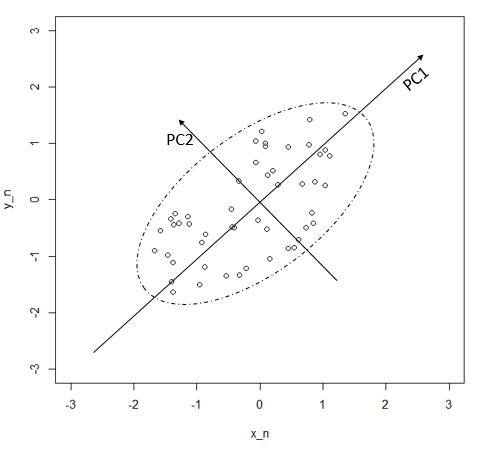## 2. 信息增益算法及其在化学信息学中的应用

### 2.1 信息增益介绍

$$Entropy(s) = -p1 * Log2(p1) - p2 * Log2(p2)$$

$$Entropy(T) = Pt * Entropy(t) + (Pt’) * entropy(t’)$$

$$IG(T) = Entropy(s) - Entropy(T)$$

### 2.2 信息增益在化学信息学中的应用

#### 决策树分类模型构建## 3. 主成分分析方法及其在化学信息学中的应用

### 3.1 主成分分析算法简介

1）对原始数据进行标准化即$x ̃(i,j) = [ x(i,j) - x*(j) ] / s(j)$，其中x*(j)是第j列变量的平均数。这样X ̃中每列的变量都满足平均数为0方差为1。这样的好处是所有描述符的方差都一样，进一步计算协方差阵、相关阵会变得非常容易，且计算本身并没有丢失变量本身的价值。
2）计算相关矩阵即$R = X ̃^T X/(n-1)(R(j,j’) = Σi[x ̃(i,j) * x ̃(i,j’)] / (n-1))$，相关阵可以理解 R(j,j’)即第j个描述符与第j’个描述符的皮尔逊相关系数。
3）对R进行特征分解 R = UAUT，其中A = diag(λ1，λ2…)，λ 即为 R 的特征值。求解时要求 λ1>λ2>… 。U是每个描述符的特征向量，第j列即第j个描述符的特征向量。
4）用特征向量描述原变量，即 ξ(j) = U(j)Y，这里的Y即主成分。可以把主成分想象成一个新的描述符组，那么U就是让主成分映射到原描述符的变化矩阵。上式还可以简化为 ξ = UY。 由于U是正交阵，所以可以得到Y = Uξ，即可以从原描述符组ξ映射到主成分Y。
5）计算 (λ1+λ2+λ3+…+λj) / Σλ 得到前j个主成分的贡献率来判断要选取几个主成分。假设原来有10描述符即可以得到10个主成分，但一般我们可能会发现前5个主成分已经有80%的贡献率，那么我们可以选择前5个主成分作为描述符变量，这样成功的缩小一般的变量数，而“信息量”并没有损失太多。

### 3.2 主成分原理及其几何意义简介

y1 = x1 cos(θ) + x2 sin(θ)
y2 = -x1 sin(θ) + x2cos(θ)## 参考文献

 Brown F K. . Chemoinformatics: What is it and How does it Impact Drug Discovery[J]. Annual reports in medicinal chemistry, 1998, 33: 375-384.
 Hansch C, Fujita T. p-σ-π Analysis. A method for the correlation of biological activity and chemical structure[J]. Journal of the American Chemical Society, 1964, 86(8): 1616-1626.
 Free S M, Wilson J W. A mathematical contribution to structure-activity studies[J]. Journal of Medicinal Chemistry, 1964, 7(4): 395-399.
 Shen J, Cheng F, Xu Y, et al. Estimation of ADME properties with substructure pattern recognition[J]. Journal of chemical information and modeling, 2010, 50(6): 1034-1041.
 刘建平, 朱坤平, 陆元鸿. 应用数理统计[M]. 华东理工大学出版社. ISBN: 978-7-5628-3264-5/O·244
 汪冬化. 多元统计分析与SPSS应用[M]. 华东理工大学出版社. ISBN: 978-7-5628-2874-7/F·228
 Owen J R, Nabney I T, Medina-Franco J L, et al. Visualization of molecular fingerprints[J]. Journal of chemical information and modeling, 2011, 51(7): 1552-1563.
 Kong L, Kadokami K, Wang S, et al. Monitoring of 1300 organic micro-pollutants in surface waters from Tianjin, North China[J]. Chemosphere, 2014.
 Xiao X, Zeng X, Yuan Y, et al. Understanding the conformation transition in the activation pathway of [small beta]2 adrenergic receptor via a targeted molecular dynamics simulation [J]. Physical Chemistry Chemical Physics, 2015,
 Chen J, Liang Z, Wang W, et al. Revealing Origin of Decrease in Potency of Darunavir and Amprenavir against HIV-2 relative to HIV-1 Protease by Molecular Dynamics Simulations [J]. Sci Rep, 2014, 4